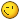## Reply: Does any one have any idea how to approach this PERT question?

 Name E-mail Your e-mail address will never be displayed on the site. Subject Message [quote="Gabriella Dellino, PMP" post=22632]Wow, Ali! That truly is a tough one! Let me recap the assumptions here: 1. Two activities on the critical path 2. Activity duration following a Beta distribution 3. Duration uncertainty (i.e., standard deviation, sigma) known for each activity. Please notice that the description here sounds a bit misleading to me. In fact, if you assume a beta distribution, the standard deviation is sigma = (P - O)/6, where P and O are the most pessimistic and most optimistic durations, respectively. Not that we will use this formula to solve this problem, but reading it as P - O confused me in the beginning. 4. Confidence interval as +/- 3sigma. This won't be needed to solve our problem ;) Now, from some statistical theorems, we know that the variance of the project is equal to the sum of the variances of the activities on the critical path. In our case: var_p = var_1 + var_2 = (sigma_1)^2 + (sigma_2)^2 = 18^2 + 24^2 = 900 So the standard deviation for the project (i.e., its duration uncertainty) is sigma_p = sqrt(var_p) = 30. Therefore the correct answer should be option B. That said, I've never seen anything like this in the actual exam, nor even in the exam simulations I practiced. Thanks for sharing it. Gabriella[/quote]

## Topic History of : Does any one have any idea how to approach this PERT question?

Max. showing the last 6 posts - (Last post first)
1 year 3 months ago #22649Awesome !Good job
Thanks very much Gabriella
1 year 3 months ago #22632

Gabriella Dellino, PMPWow, Ali! That truly is a tough one!

Let me recap the assumptions here:
1. Two activities on the critical path
2. Activity duration following a Beta distribution
3. Duration uncertainty (i.e., standard deviation, sigma) known for each activity.
Please notice that the description here sounds a bit misleading to me. In fact, if you assume a beta distribution, the standard deviation is sigma = (P - O)/6, where P and O are the most pessimistic and most optimistic durations, respectively. Not that we will use this formula to solve this problem, but reading it as P - O confused me in the beginning.
4. Confidence interval as +/- 3sigma. This won't be needed to solve our problemNow, from some statistical theorems, we know that the variance of the project is equal to the sum of the variances of the activities on the critical path. In our case:
var_p = var_1 + var_2 = (sigma_1)^2 + (sigma_2)^2 = 18^2 + 24^2 = 900
So the standard deviation for the project (i.e., its duration uncertainty) is sigma_p = sqrt(var_p) = 30.

Therefore the correct answer should be option B.

That said, I've never seen anything like this in the actual exam, nor even in the exam simulations I practiced. Thanks for sharing it.

Gabriella
1 year 3 months ago #22623Together with your team, you applied three-point estimation on a critical path
which consists of two activities.
The following duration uncertainties are all calculated assuming a ±3sigma
confidence interval.
The duration uncertainty—defined as pessimistic minus optimistic estimate—of
the first activity is 18 days; the second estimate has an uncertainty of 24 days.
Applying the PERT formula for paths (beta distribution), what is the duration
uncertainty of the entire path?

o 21 days
o 30 days
o 42 days
o No statement is possible from the information given
FROM OLIVER LEMANN FREE SAMPLES

OSP INTERNATIONAL LLC
OSP INTERNATIONAL LLC
Training for Project Management Professional (PMP)®, PMI Agile Certified Practitioner (PMI-ACP)®, and Certified Associate in Project Management (CAPM)®